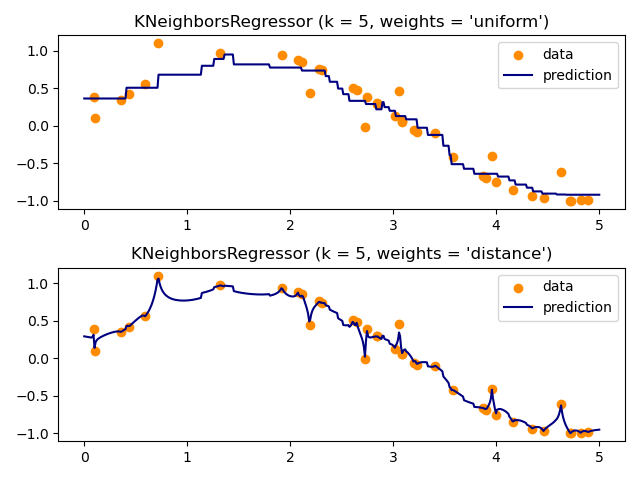# Nearest Neighbors regression¶

Demonstrate the resolution of a regression problem using a k-Nearest Neighbor and the interpolation of the target using both barycenter and constant weights.

# Author: Alexandre Gramfort <alexandre.gramfort@inria.fr>
#         Fabian Pedregosa <fabian.pedregosa@inria.fr>
#
# License: BSD 3 clause (C) INRIA


## Generate sample data¶

import numpy as np
import matplotlib.pyplot as plt
from sklearn import neighbors

np.random.seed(0)
X = np.sort(5 * np.random.rand(40, 1), axis=0)
T = np.linspace(0, 5, 500)[:, np.newaxis]
y = np.sin(X).ravel()

y[::5] += 1 * (0.5 - np.random.rand(8))


## Fit regression model¶

n_neighbors = 5

for i, weights in enumerate(["uniform", "distance"]):
knn = neighbors.KNeighborsRegressor(n_neighbors, weights=weights)
y_ = knn.fit(X, y).predict(T)

plt.subplot(2, 1, i + 1)
plt.scatter(X, y, color="darkorange", label="data")
plt.plot(T, y_, color="navy", label="prediction")
plt.axis("tight")
plt.legend()
plt.title("KNeighborsRegressor (k = %i, weights = '%s')" % (n_neighbors, weights))

plt.tight_layout()
plt.show()Total running time of the script: ( 0 minutes 0.186 seconds)

Gallery generated by Sphinx-Gallery